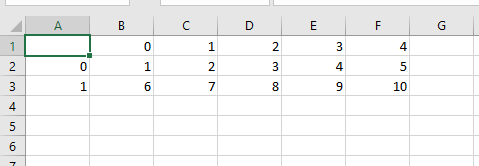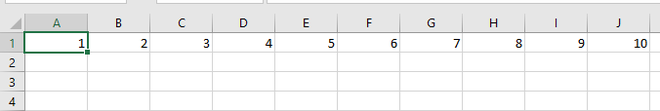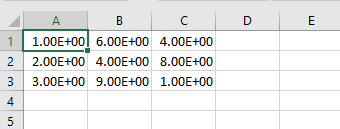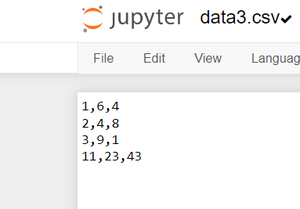Open in App
Not now

# Convert a NumPy array into a csv file

• Last Updated : 30 Sep, 2022

In this article, we are going to see different methods to save a NumPy array into a CSV file. CSV file format is the easiest and useful format for storing data

There are different methods by which we can save the NumPy array into a CSV file

## Convert a NumPy array into a CSV using Dataframe.to_csv()

This method is used to write a Dataframe into a CSV file. Converting the array into pandas Dataframe and then saving it to CSV format.

## Python3

 `# import necessary libraries` `import` `pandas as pd` `import` `numpy as np`   `# create a dummy array` `arr ``=` `np.arange(``1``,``11``).reshape(``2``,``5``)`   `# display the array` `print``(arr)`   `# convert array into dataframe` `DF ``=` `pd.DataFrame(arr)`   `# save the dataframe as a csv file` `DF.to_csv(``"data1.csv"``)`

Output:## Convert a NumPy array into a CSV using numpy_array.tofile()

This method is used to write an array into the file. Create an array then save it into a CSV file.

## Python3

 `# import the necessary library` `import` `numpy as np`   `# create a dummy array` `arr ``=` `np.arange(``1``,``11``)`   `# display the array` `print``(arr)`   `# use the tofile() method ` `# and use ',' as a separator` `# as we have to generate a csv file` `arr.tofile(``'data2.csv'``, sep ``=` `','``)`

Output:## Convert a NumPy array into a CSV using numpy.savetext()

This method is used to save an array to a text file. Create an array then save it as a CSV file.

## Python3

 `# import numpy library` `import` `numpy`   `# create an array` `a ``=` `numpy.array([[``1``, ``6``, ``4``],` `                 ``[``2``, ``4``, ``8``],` `                 ``[``3``, ``9``, ``1``]])`   `# save array into csv file` `numpy.savetxt(``"data3.csv"``, a, ` `              ``delimiter ``=` `","``)`

Output:## Convert a NumPy array into a CSV using file handling

A formatter inserts one or more replacement fields and placeholders into a string by using the str.format function and a pair of curly braces (). The value will be inserted into the placeholders and joined to the string that is supplied as input to the format function. The with is used to write in the CSV file.

## Python3

 `# import numpy library` `import` `numpy`   `# create an array` `a ``=` `numpy.array([[``1``, ``6``, ``4``],` `                ``[``2``, ``4``, ``8``],` `                ``[``3``, ``9``, ``1``], ` `                ``[``11``, ``23``, ``43``]])`   `# save array into csv file` `rows ``=` `[``"{},{},{}"``.``format``(i, j, k) ``for` `i, j, k ``in` `a]` `text ``=` `"\n"``.join(rows)`   `with ``open``(``'data3.csv'``, ``'w'``) as f:` `    ``f.write(text)`

Output:My Personal Notes arrow_drop_up
Related Articles